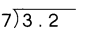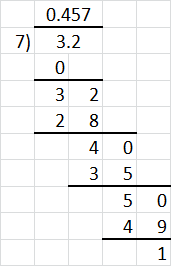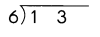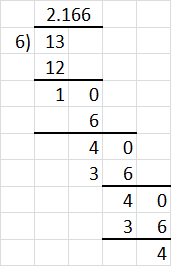# Math in Focus Grade 5 Chapter 9 Practice 3 Answer Key Dividing Decimals

Practice the problems of Math in Focus Grade 5 Workbook Answer Key Chapter 9 Practice 3 Dividing Decimals to score better marks in the exam.

## Math in Focus Grade 5 Chapter 9 Practice 3 Answer Key Dividing Decimals

Divide. Write the quotient as a decimal.

Example
0.6 ÷ 2 = 6 tenths ÷ 2 tenths
= 3 tenths
= 0.3
So, 0.6 ÷ 2 = 0.3

Question 1.
0.8 ÷ 4 = ___ tenths ÷ 4
= ___ tenths
= ___
So, 0.8 ÷ 4 = ___
Explanation:
0.8 ÷ 4 = 8 tenths ÷ 4
= 2 tenths
= 0.2
So, 0.8 ÷ 4 = 0.2

Question 2.
1 ÷ 5 = ___ tenths ÷ 5
= ___ tenths
= ___
So, 1 ÷ 5 = ___
Explanation:
1 ÷ 5 = _10__ tenths ÷ 5
= 2 tenths
= 0.2
So, 1 ÷ 5 = 0.2

Question 3.
2.4 ÷ 6 = ___ tenths ÷ 6
= ___ tenths
= ___
So, 2.4 ÷ 6 = ___
Explanation:
2.4 ÷ 6 = 24 tenths ÷ 6
= 4 tenths
= 0.4
So, 2.4 ÷ 6 =0.4

Complete. Write the quotient as a decimal.

Example
0.08 ÷ 2 = 8 hundredths ÷ 2
= 4 hundredths
= 0.04
So, 0.08 ÷ 2 = 0.04.

Question 4.
0.14 ÷ 7 = ________ hundredths ÷ ________
= _________ hundredths
= _____
So, 0.14 ÷ 7 = _____
Explanation:
0.14 ÷ 7 = 14 hundredths ÷ 7
=2 hundredths
= 0.02
So, 0.14 ÷ 7 = 0.02

Question 5.
0.27 ÷ 9 ________ hundredths ÷ ________
= _________ hundredths
= _____
So, 0.27 ÷ 9 = _____
Explanation:
0.27 ÷ 9 = 27 hundredths ÷ 9
= 3 hundredths
= 0.03
So, 0.27 ÷ 9 = 0.03

Question 6.
0.1 ÷ 2 ________ hundredths ÷ ________
= _________ hundredths
= _____
So, 0.1 ÷ 2 = _____
Explanation:
0.1 ÷ 2 = 10 hundredths ÷ 2
= 5 hundredths
=0.05
So, 0.1 ÷ 2 = 0.05

Follow the steps to divide 8.4 by 3. Fill in the blanks.

Question 7.
Step 1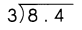Divide the ones by 3.
8 ones ÷ 3 = __ ones R __ ones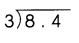Regroup the remainder into tenths.
___ ones = ___ tenths
__ tenths + 4 tenths = ___ tenths

Step 2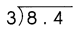Divide the tenths by 3.
__ tenths ÷ 3 = ___ tenths
So, 8.4 ÷ 3 = ___
Explanation:
Step 1Divide the ones by 3.
8 ones ÷ 3 = 2 ones R 2 onesRegroup the remainder into tenths.
2 ones = 20 tenths
20 tenths + 4 tenths = 24 tenths

Step 2Divide the tenths by 3.
24 tenths ÷ 3 = 8 tenths
So, 8.4 ÷ 3 = 2.8

Divide.

Question 8.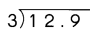Explanation:
Step 1Divide the ones by 3.
12 ones ÷ 3 = 4 ones R 0 onesRegroup the remainder into tenths.
0 ones = 0 tenths
0 tenths + 9 tenths = 9 tenths

Step 2Divide the tenths by 3.
9 tenths ÷ 3 = 3 tenths
So, 12.9 ÷ 3 = 4.3

Question 9.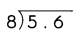Explanation:
Step 1Divide the ones by 8.
5.6 x 10 = 56
56 ones ÷ 8 = 7 ones R 0 onesRegroup the remainder into tenths.
7 ones = 7 tenths
0 tenths + 7 tenths = 7 tenths
So, 5.6 ÷ 8 = 0.7

Question 10.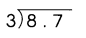Explanation:
Step 1Divide the ones by 3.
8.7 x 10 = 87
87 ones ÷ 3 = 29 ones R 0 onesRegroup the remainder into tenths.
29 = 2 ones and 9 tenths
2 tenths + 9 tenths = 9 tenths
So, 5.6 ÷ 8 = 2.9

Question 11.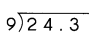Explanation:
Step 1Divide the ones by 9.
24 ones ÷ 9 = 2 ones R 6 onesRegroup the remainder into tenths.
26 = 2 ones and 6 tenths
Step 2Divide the tenths by 9.
3 tenths ÷ 9 = 27tenths
So, 24.3 ÷ 9 = 2.7

Question 12.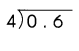Explanation:
Step 1Divide the ones by 4.
0.6 x 10 = 6
6 ones ÷ 4 = 1 ones R 2onesRegroup the remainder into tenths.
1 ones = 1 tenths
4 tenths + 7 1tenths = 5 tenths
So, 0.6 ÷ 4 = 0.15

Question 13.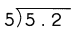Explanation:
Step 1Divide the ones by 5.
5.2 x 10 = 52
52 ones ÷ 5 = 1 ones R 4 onesRegroup the remainder into tenths.
104 = 1 ones and 4 tenths
1 tenths + 4 tenths = 104 tenths
So, 5.2 ÷ 5 = 1.04

Follow the steps to divide 5.48 by 4. Fill in the blanks.

Question 14.
Step 1.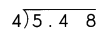Divide the ones by 4.
5 ones ÷ 4 = ______ one R ___ one
Regroup the remainder into tenths.
__ one = ___ tenths
_________ tenths + 4 tenths = _________ tenths

Step 2.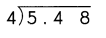Divide the tenths by 4.
_________ tenths ÷ 4 _________ tenths R _________ tenths
Regroup the remainder into hundredths.
_________ tenths = _________ hundredths
_________ hundredths + 8 hundredths = ________ hundredths

Step 3.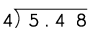Divide the hundredths by 4.
_________ hundredths ÷ 4 = _________ hundredths
So, 5.48 ÷ 4 = _____
Explanation:
Step 1.Divide the ones by 4.
5 ones ÷ 4 = 1 one R 1 one
Regroup the remainder into tenths.
1 one = 10 tenths
10 tenths + 4 tenths = 14 tenths

Step 2.Divide the tenths by 4.
14 tenths ÷ 4
3 tenths R 2 tenths
Regroup the remainder into hundredths.
2 tenths = 20 hundredths
20 hundredths + 8 hundredths = 28 hundredths

Step 3.Divide the hundredths by 4.
28 hundredths ÷ 4 = 7 hundredths
So, 5.48 ÷ 4 = 1.37

Divide.

Question 15.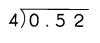Explanation:
Step 1.Divide the ones by 4.
0 ones ÷ 4 = 0 one R  one
Regroup the remainder into tenths.
0 one = 0 tenths
0 tenths + 5 tenths = 5 tenths

Step 2.Divide the tenths by 4.
5 tenths ÷ 4
1 tenths R 1 tenths
Regroup the remainder into hundredths.
1 tenths = 10 hundredths
10 hundredths + 2 hundredths = 12 hundredths

Step 3.Divide the hundredths by 4.
12 hundredths ÷ 4 = 3 hundredths
So, 0.52 ÷ 4 = 0.13

Question 16.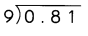Explanation:
Step 1.Divide the ones by 9.
0 ones ÷ 9 = 0 one R  one
Regroup the remainder into tenths.
0 one = 0 tenths
0 tenths + 8 tenths = 8 tenths

Step 2.Divide the tenths by 9
8 tenths ÷ 9
0 tenths R 8 tenths
Regroup the remainder into hundredths.
8 tenths = 80 hundredths
80 hundredths + 1 hundredths = 81 hundredths

Step 3.Divide the hundredths by 9.
81 hundredths ÷ 9 = 9 hundredths
So, 0.81 ÷ 9 = 0.09

Question 17.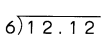Explanation:
Step 1.Divide the ones by 6
12 ones ÷ 6 = 2 one R  0 one
Regroup the remainder into tenths.
2 one = 0 tenths
0 tenths +1 tenths = 1 tenths

Step 2.Divide the tenths by 9
1 tenths ÷ 9
1 tenths R 2 tenths
Regroup the remainder into hundredths.
1 tenths = 10 hundredths
10 hundredths + 2 hundredths = 12 hundredths

Step 3.Divide the hundredths by 9.
12 hundredths ÷ 6 = 2 hundredths
So, 12.12 ÷ 6 = 2.02

Question 18.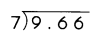Explanation:
Step 1.Divide the ones by 7
9 ones ÷ 7 = 1 one R 2 one
Regroup the remainder into tenths.
2 one = 20 tenths
20 tenths + 6 tenths = 26 tenths

Step 2.Divide the tenths by 7
26 tenths ÷ 7
3 tenths R 5 tenths
Regroup the remainder into hundredths.
5 tenths = 50 hundredths
60 hundredths + 6 hundredths = 56 hundredths

Step 3.Divide the hundredths by 7
56 hundredths ÷ 7 = 8 hundredths
So, 9.66 ÷ 7 = 1.38

Question 19.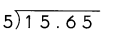Explanation:
Step 1.Divide the ones by 5.
15 ones ÷ 5 = 3 one R 0 one
Regroup the remainder into tenths.
0 one = 0 tenths
0 tenths + 6 tenths = 6 tenths

Step 2.Divide the tenths by 5.
6 tenths ÷ 5
1 tenths R 1 tenths
Regroup the remainder into hundredths.
1 tenths = 10 hundredths
10 hundredths + 5 hundredths = 15 hundredths

Step 3.Divide the hundredths by 5.
15 hundredths ÷ 5 = 3 hundredths
So, 15.65 ÷ 5 = 3.13

Question 20.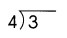3 ÷ 4 = 3 tenths ÷ 4
= 30 tenths
30 ÷ 4 = 7 R 2
Divide the tenths by 4.
20 tenths ÷ 4
Regroup the remainder into hundredths.
2 tenths = 20 hundredths
20 ÷ 4 = 5 hundredths
So, 3 ÷ 4 = 0.75

Divide. Round each quotient to the nearest tenth.

Example
7 ÷ 8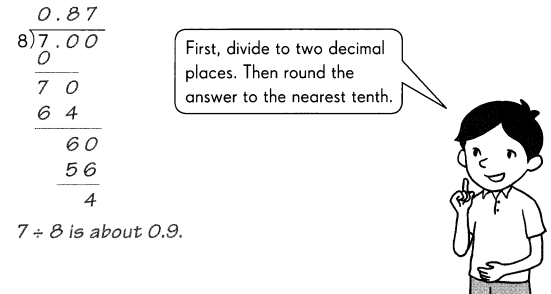Question 21.
5 ÷ 7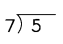Explanation:
First, divide to two decimal places.
Then round the answer to the nearest tenth.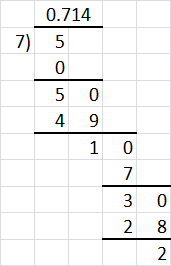Question 22.
11 ÷ 9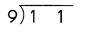Explanation:
First, divide to two decimal places.
Then round the answer to the nearest tenth.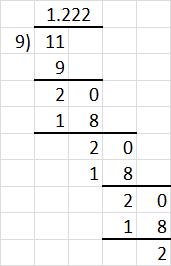11 ÷ 9 is about 1.222

Divide. Round each quotient to the nearest hundredth.

Example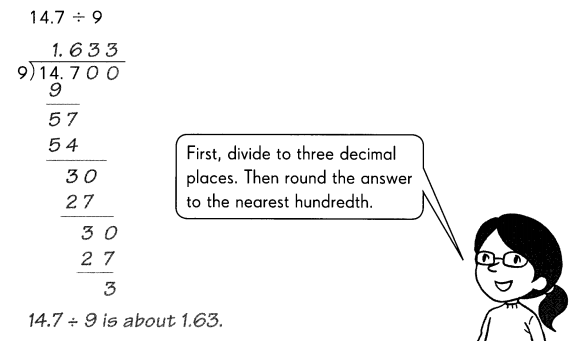Question 23.
3.2 ÷ 7